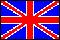ISSN 2079-3537

Scientific Visualization
 Issue Year: 2012 Quarter: 2 Volume: 4 Number: 2 Pages: 1 - 13
 Article Name: MULTIDIMENSIONAL DATA ANALYSIS FOR MULTIPARAMETRIC OPTIMIZATION PROBLEMS USING VISUALIZATION METHODS Authors: A. Bondarev (Russia), V. Galaktionov (Russia) Address: A. Bondarev bond@keldysh.ru Keldysh Institute for Applied Mathematics RAS, Moscow, Russia   V. Galaktionov vlgal@gin.keldysh.ru Keldysh Institute for Applied Mathematics RAS, Moscow, Russia Abstract: The problems of optimizing analysis have high priority at the moment due to multiprocessor computers and parallel computations. Optimizing analysis is based on mass solution of inverse problems. At the same time the determining parameters are changed in definite ranges for the class of problem under consideration. The results of such computations are as multidimensional arrays. Dimensionality of such array corresponds to the number of determining parameters. These arrays need processing and visual presentation for data analysis. Therefore, one needs common scheme for data processing. The paper considers the problems of multidimensional numerical data processing and analysis. The volumes of numerical data are written in a form of multidimensional arrays. The data are considered as numerical solutions of CFD inverse problems and optimization problems. The numerical results are obtained by parallel computations. Using visualization methods common scheme of data processing and analysis is described. The scheme is applied to the practical problem of the space-time structures localization for interacting time-dependent flows. Language: Russian